VNWA3E has special functions to extract the lumped elements parameters of circuits or some components.  Today, I will explain how to use this functions with the following 1 [nF] ceramic capacitor.First, I measured the S11 parameters and other parameters using the special tool which is designed for through-hole components.  We can easily expect that component wire produces parasitic inductance, which results in some resonance at some frequency.The following figure shows the measurement results. The frequency range is from 1 MHz to 60 MHz. The blue line is S11 [dB]. The Yellow line is capacitance value which is calculated from S11. The magenta line is  real value of the impedance. We can see that there is a resonant around 30~40 MHz.The equivalent circuit of this capacitor can be expressed as the combination of the capacitance and parasitic inductance and internal resistance.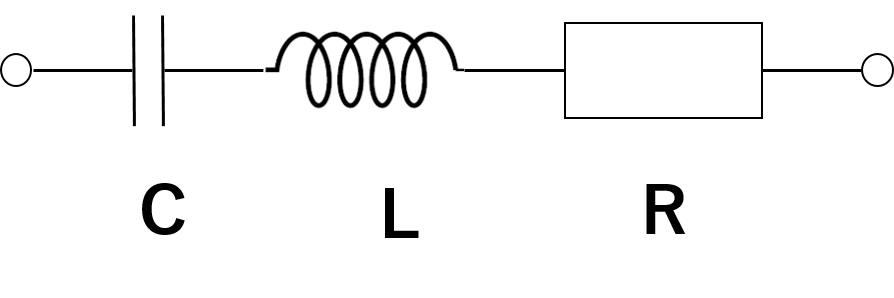Open the optimizer window. (VNWA3E program, tool menu – optimizer), input the parameters as the following figure.

We know that C is around 1 [nF]  and R is around 0.15 [Ohm] from the measured value. and I assumed that L is around 10 [nH].

Then click the optimize button in the menu bar.The calculated fitted results is shown like this figure.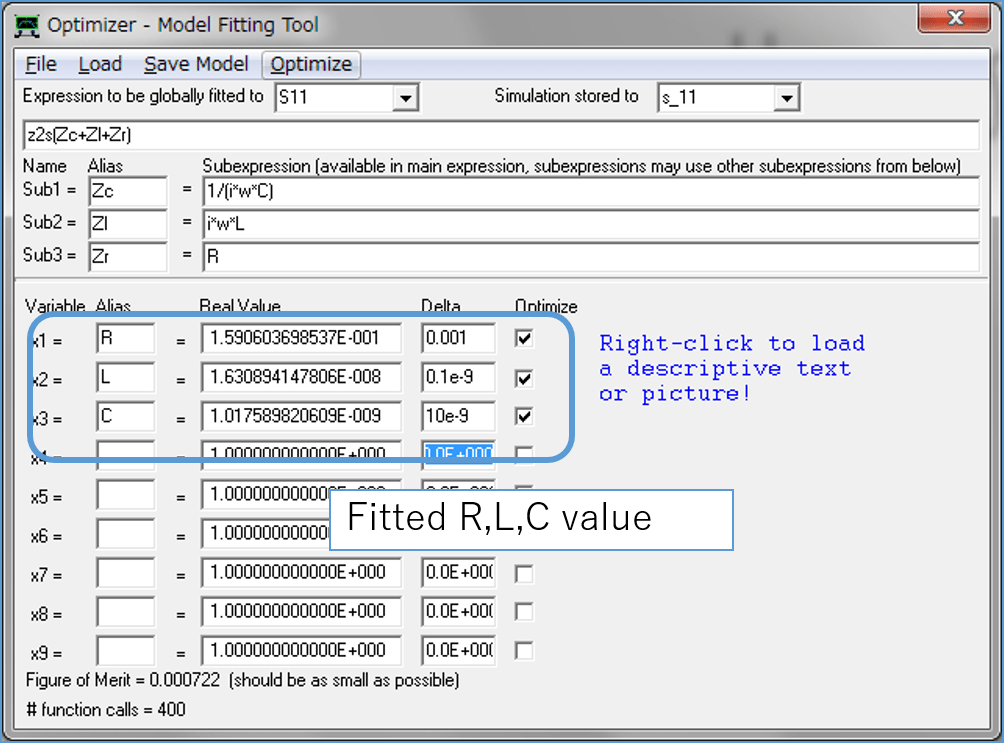That means that the equivalent circuit of the capacitor is like the following figure.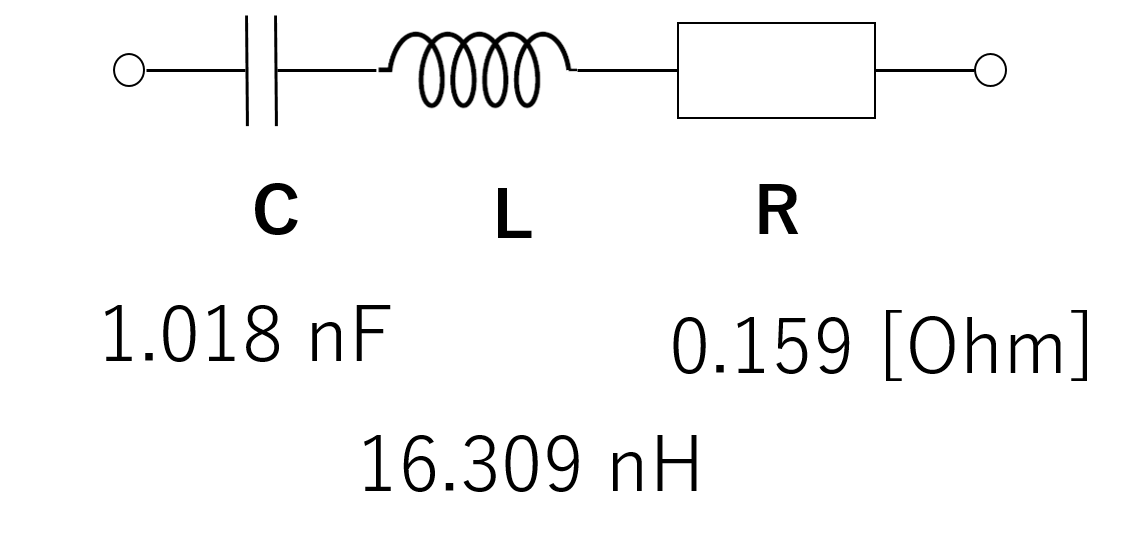In order to check the feasibility of these value, We will plot the simulated value with this fitted data over the measured value.

In this process, We are using the custom trace menu. You can access the custom trace menu like this picture.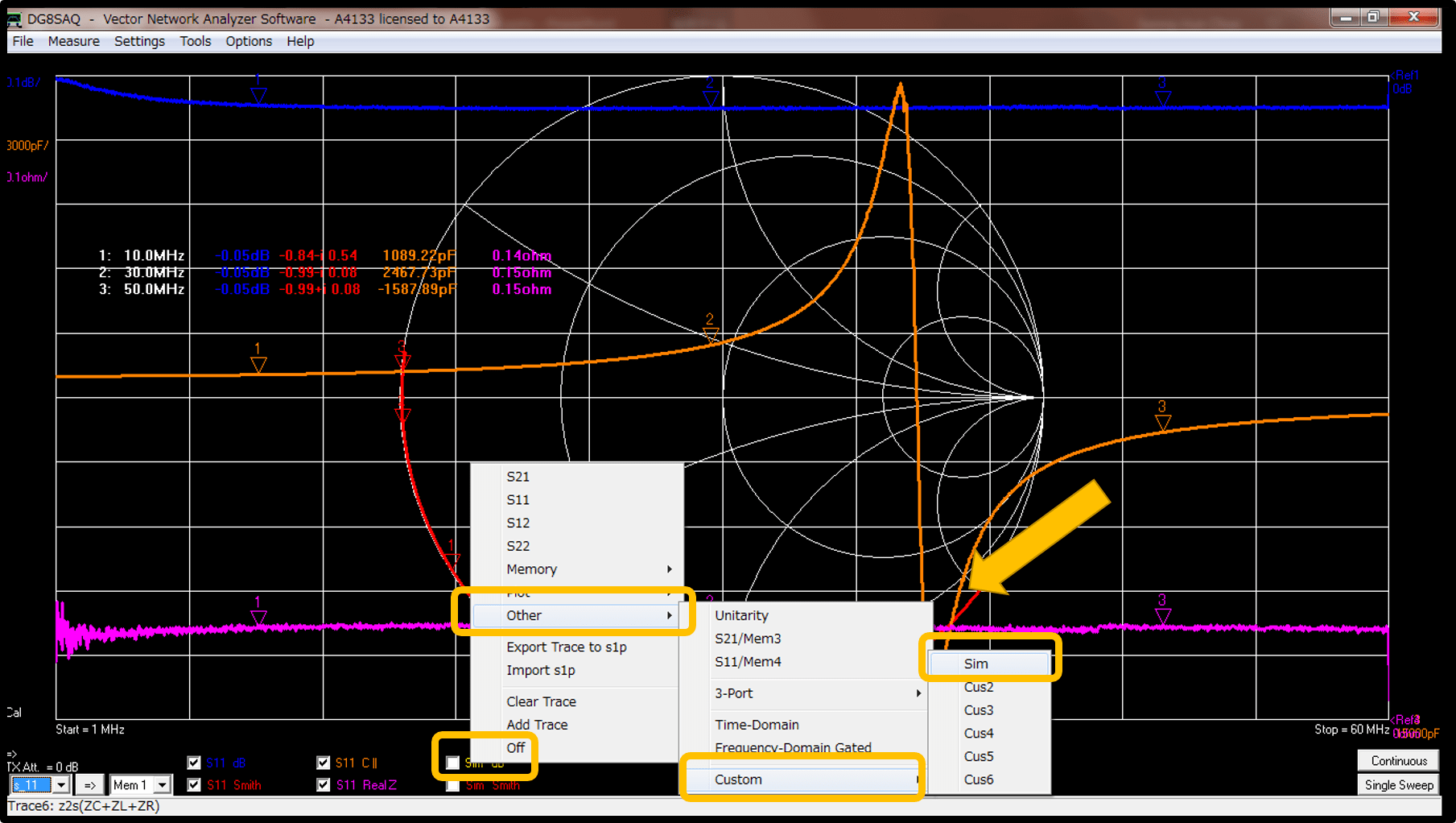Then input the related equations and values like this.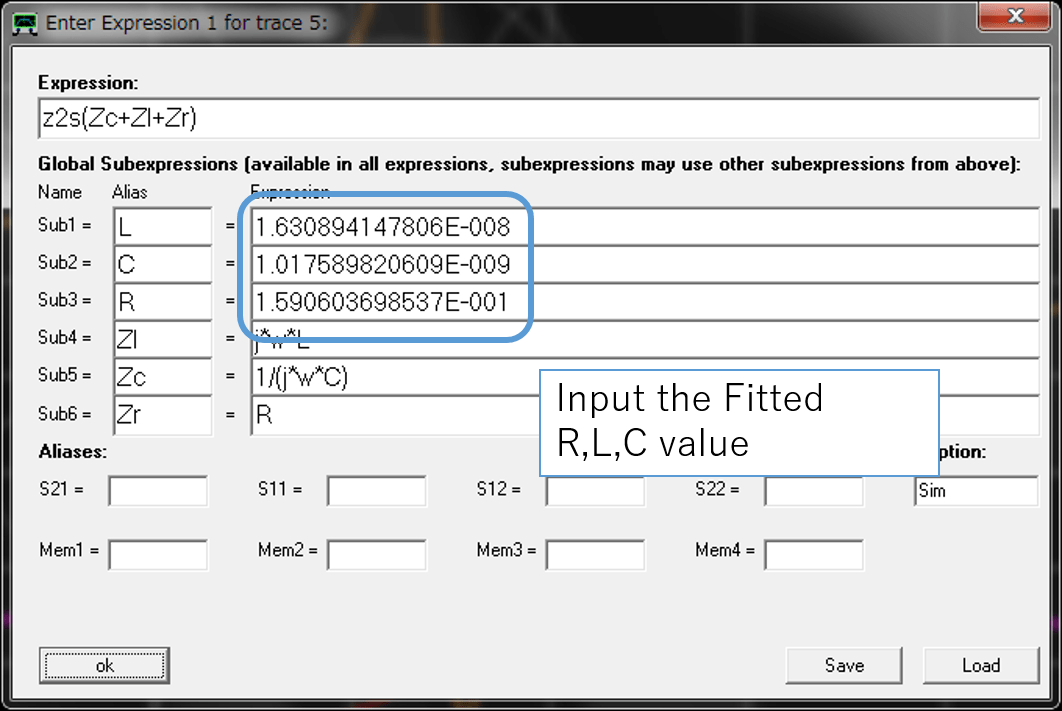Then Check the results.

(1) S11 dB(2) Capacitance(3) Smith ChartWe can see the simulated results matched well with the measured results.

It was really amazing that we can extract the lumped elements parameters using only one measurement with VNWA.  Next time, I will show how to extract the lumped elements parameters of the WPT coils by using this method.

## 1 Comment

1. […]  http://wireless-square.com/2016/11/10/extraction-of-lumped-elements-parameters-using-vnwa/ […]

このサイトはスパムを低減するために Akismet を使っています。コメントデータの処理方法の詳細はこちらをご覧ください